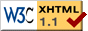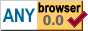The Deadbeef Random Number Generator

# The Deadbeef Random Number Generator

2019-05-25

## Introduction

The deadbeef random number generator is a simple pseudorandom number generator (PRNG) that generates 32-bit pseudorandom numbers, with heavy use of the constant `0xdeadbeef`. It's simple enough that one can learn the code by heart, and implement it whenever a simple PRNG is needed. The generator is fast and does well on a number of tests I've put it through, but I make no claims about the suitability of the generated numbers for any purpose. Results of analysis and suggestions for improvement are welcome.

Note: If you are looking for a pseudorandom number generator for 8-bit systems, I suggest visiting Micrornd. It describes a PRNG specifically designed for that purpose, and has links to a few others.

## C Code

Below is the C code for the deadbeef random number generator. Feel free to use the code in your own software. Attributing the code to me and sharing any improvements you make would, of course, be appreciated.

```#include "deadbeef_rand.h" static uint32_t deadbeef_seed; static uint32_t deadbeef_beef = 0xdeadbeef; uint32_t deadbeef_rand() { deadbeef_seed = (deadbeef_seed << 7) ^ ((deadbeef_seed >> 25) + deadbeef_beef); deadbeef_beef = (deadbeef_beef << 7) ^ ((deadbeef_beef >> 25) + 0xdeadbeef); return deadbeef_seed; } void deadbeef_srand(uint32_t x) { deadbeef_seed = x; deadbeef_beef = 0xdeadbeef; } ```

And here is a header file you can use with it:

```#ifndef _DEADBEEF_RAND_H #define _DEADBEEF_RAND_H #include <inttypes.h> uint32_t deadbeef_rand(); void deadbeef_srand(uint32_t x); #endif /* ndef _DEADBEEF_RAND_H */ ```

## Other Languages

Here are some implementations of the deadbeef pseudo-random number generator in languages other than C:

The tests below have been carried out using the C implementation given above, but the implementations in other languages yield the same results when initialized with the same seed.

## Performance

In an effort to establish if the deadbeef random number generator is any good, I've run it through a number of test programs. I would like to emphasize that I do not consider myself to have enough knowledge of statistics to do a proper analysis of pseudorandom number generators, so, please, do not attach too much importance to these results.

### The Image Test

First, I generated a file containing 223 (8388608) numbers generated by deadbeef. This took 3.24 seconds. For comparison: `rand()` from glibc took 4.60 seconds to perform the same task.

Then, I fed the file to an image generator, which generates images that give an indication of how uniformly the generated numbers are distributed. The image conists of two 256 by 256 pixel areas that are initially black. Each random number is broken up in its lower 16 bits and its higher 16 bits, and each of these parts is used as an index in the image: the lower 16-bits are used as an index in the upper part of the image, and the higher 16-bits are used as an index in the upper part of the image. The two pixels designated in this way are increased in intensity. For a uniformly distributed sample, this results in a uniform, grey image.

This is the image generated for the deadbeef random number generator (note: the translucency of the page body causes the background image to show through the image. Click here to view the image by itself):As you can see, it's pretty uniformly grey. This is good.

### The Ones Test

Next, I counted the number of times each bit in the generated numbers was 1, and divided this by the number of numbers to get the probability of each bit being a 1. These probabilities are on an interval from 0 to 1, where 0 means the bit is always 0, and 1 means it's always a 1. The results are as follows:

```bit 0: 0.500188
bit 1: 0.499962
bit 2: 0.499928
bit 3: 0.500084
bit 4: 0.500149
bit 5: 0.500065
bit 6: 0.500234
bit 7: 0.500105
bit 8: 0.499910
bit 9: 0.499993
bit 10: 0.500327
bit 11: 0.499843
bit 12: 0.499985
bit 13: 0.499863
bit 14: 0.499945
bit 15: 0.500151
bit 16: 0.499762
bit 17: 0.500145
bit 18: 0.499812
bit 19: 0.499892
bit 20: 0.500206
bit 21: 0.499900
bit 22: 0.499934
bit 23: 0.500006
bit 24: 0.500333
bit 25: 0.500159
bit 26: 0.500011
bit 27: 0.500134
bit 28: 0.500053
bit 29: 0.500149
bit 30: 0.500236
bit 31: 0.499856
```

Since all probabilities are very close to 0.5, there is about an equal chance that any bit in a generated number will be a 1 or a 0. This is good.

### The Predict-Next Test

As a final test, I had a program compute the correlations between bits in subsequent numbers. For each bit in the current number and each bit in the previous number, the program calculates the probability that they are the same. This is scaled on an interval from -1 to 1, where -1 means that the two bits are always complementary, and 1 means they are always identical. The program prints the 32 strongest correlations (furthest from 0).

```From bit 8 to bit 12: 0.001018
From bit 23 to bit 13: 0.000996
From bit 4 to bit 11: -0.000983
From bit 22 to bit 26: -0.000975
From bit 12 to bit 22: 0.000970
From bit 14 to bit 21: -0.000915
From bit 21 to bit 28: 0.000915
From bit 11 to bit 18: 0.000905
From bit 18 to bit 25: -0.000903
From bit 25 to bit 0: 0.000903
From bit 17 to bit 24: -0.000899
From bit 22 to bit 10: 0.000898
From bit 24 to bit 31: 0.000898
From bit 10 to bit 17: -0.000888
From bit 29 to bit 11: 0.000884
From bit 5 to bit 7: -0.000882
From bit 17 to bit 20: 0.000868
From bit 8 to bit 15: -0.000867
From bit 20 to bit 31: -0.000867
From bit 22 to bit 29: -0.000854
From bit 15 to bit 22: -0.000853
From bit 27 to bit 9: -0.000846
From bit 5 to bit 18: 0.000832
From bit 24 to bit 28: 0.000826
From bit 14 to bit 24: -0.000826
From bit 9 to bit 21: 0.000823
From bit 19 to bit 21: -0.000817
From bit 10 to bit 14: -0.000815
From bit 7 to bit 19: 0.000813
From bit 4 to bit 5: -0.000807
From bit 7 to bit 9: -0.000801
From bit 6 to bit 17: 0.000799
```

Since all of these numbers are fairly close to 0, it's very hard to predict the next number deadbeef will generate based on the current one. This is good.

## rngtest Results

Below is the output of rngtest (from rng-tools). There are many successes and few failures, and the scores for monobit, poker, and the runs are low, which is good.

```rngtest: starting FIPS tests...
rngtest: entropy source drained
rngtest: bits received from input: 268435456
rngtest: FIPS 140-2 successes: 13410
rngtest: FIPS 140-2 failures: 11
rngtest: FIPS 140-2(2001-10-10) Monobit: 0
rngtest: FIPS 140-2(2001-10-10) Poker: 3
rngtest: FIPS 140-2(2001-10-10) Runs: 2
rngtest: FIPS 140-2(2001-10-10) Long run: 6
rngtest: FIPS 140-2(2001-10-10) Continuous run: 0
rngtest: input channel speed: (min=2.200; avg=73.040; max=4768.372)Mibits/s
rngtest: FIPS tests speed: (min=2.057; avg=17.501; max=17.960)Mibits/s
rngtest: Program run time: 18225707 microseconds
```

## Diehard Results

The file diehard.out contains the output of the Diehard Battery of Tests of Randomness. If you know how to interpret these, please let me know. I've been told that the p-values are supposed to be more or less uniformly distributed (just as much probability of being in the 0.1 through 0.2 interval as being in the 0.7 through 0.8 interval), and there shouldn't be too many of them close to 0 and close to 1. This seems to be the case in all but the operm5 and count the ones for specific bytes, and squeeze tests.